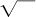NextPrevious

# Who discovered the formula for the area of a triangle?

Heron (or Hero) of Alexandria (first century B.C.E.) is best known in the history of mathematics for the formula that bears his name. This formula calculates the area of a triangle with sides a, b, and c, with s = half the perimeter: A =The Arab mathematicians who preserved and transmitted the mathematics of the Greeks reported that this formula was known earlier to Archimedes (c. 287–212 B.C.E.), but the earliest proof now known is that appearing in Heron’s Metrica.

Close

This is a web preview of the "The Handy Science Answer Book" app. Many features only work on your mobile device. If you like what you see, we hope you will consider buying. Get the App